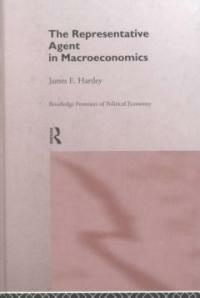> Detail View

# Detail View## The representative agent in macroeconomics (Loan 4 times)

Material type
단행본
Personal Author
Hartley, James E., 1966-
Title Statement
The representative agent in macroeconomics / James E. Hartley.
Publication, Distribution, etc
London ;   New York :   Routledge,   1997.
Physical Medium
x, 229 p. : ill. ; 24 cm.
Series Statement
Routledge frontiers of political economy ; 10
ISBN
0415146690
Bibliography, Etc. Note
Includes bibliographical references (p. 211-223) and index.
Macroeconomics -- Econometric models. Microeconomics. Econometrics. Economics -- Methodology.
 000 00979namuu2200301 a 4500 001 000001098418 005 20040510160530 008 971001s1997 enka b 001 0 eng d 020 ▼a 0415146690 035 ▼a (OCoLC)37716980 035 ▼a .b25446915 040 ▼a CU-I ▼c CU-I ▼d DLC ▼d 244002 042 ▼a lccopycat 049 0 ▼l 151158171 050 0 0 ▼a HB172.5 ▼b .H363 1997 082 0 0 ▼a 339 ▼2 21 090 ▼a 339 ▼b H332r 100 1 ▼a Hartley, James E., ▼d 1966- 245 1 4 ▼a The representative agent in macroeconomics / ▼c James E. Hartley. 260 ▼a London ; ▼a New York : ▼b Routledge, ▼c 1997. 300 ▼a x, 229 p. : ▼b ill. ; ▼c 24 cm. 440 0 ▼a Routledge frontiers of political economy ; ▼v 10 504 ▼a Includes bibliographical references (p. 211-223) and index. 650 0 ▼a Macroeconomics ▼x Econometric models. 650 0 ▼a Microeconomics. 650 0 ▼a Econometrics. 650 0 ▼a Economics ▼x Methodology.

### Holdings Information

No. Location Call Number Accession No. Availability Due Date Make a Reservation Service
No. 1 Location Call Number 339 H332r Accession No. 151158171 Availability Available Due Date Make a Reservation Service

### Contents information

```
CONTENTS
Acknowledgments = ⅹ
Part Ⅰ. Why representative agents?
1. INTRODUCTION = 3
Macroeconomics = 3
What is a representative agent model? = 4
Plan of the book = 6
2. THE ORIGINS OF THE REPRESENTATIVE AGENT = 9
The birth of the representative agent = 9
The critique and abandonment of the representative firm = 12
Modern lessons = 16
3. ARGUMENT FOR THE NEW CLASSICAL USE OF REPRESENTATIVE AGENT MODELS = 19
The return of the representative agent model = 19
The first new classical representative agent model = 20
The Lucas critique = 23
Microfoundations = 29
Discussion = 30
Part Ⅱ. The Lucas critique
4. BEYOUND TASTE AND TECHNOLOGY PARAMETERS IN MACROECONOMICS = 33
The Lucas critique = 33
Theoretical considerations = 34
An examination of Lucas(1976) = 37
An example = 40
Representative agents and the Lucas critique = 52
Is it time to dispense with the Lucas critique? = 53
5. WALRASIAN METHODOLOGY = 59
Walrasian and Marshallian traditions = 59
Walrasian methodology = 59
The role of mathematics = 62
Assumptions in Walrasian methodology = 64
The representative agent assumption = 66
The fundamental theorems? = 68
6. MARSHALLIAN METHODOLOGY = 73
Introduction = 73
Mathematics in Marsballian methodology = 75
The role of assumptions = 77
Partical equilibrium = 78
Milton Friedman = 80
The representative agent = 82
7. THE NEW CLASSICALS AS WALRASIAN ECONOMISTS = 84
The methodology of new classical economics = 84
New classical methodological statements = 84
Friedaman's comments on Lange = 91
The new classical representative agent in a Walrasian model = 101
Part Ⅳ. Microfundations
8. MICROFOUNDATIONS: AUSTRIAN STYLE = 105
Introduction = 105
Austrian methodology = 106
The Austria rejection of macroeconomics = 107
The representative agent = 113
Ideal types and representative agents = 115
9. THE TRADITIONAL CASE FOR MICROFOUNDATIONS = 120
Introduction = 120
The new classicals = 120
What are microfoundations anyway? = 127
Where does the representative agent fit in? = 130
10. THE AGGREGATION PROBLEM = 132
The general problem = 132
The basic theoretical results = 133
Extensions = 135
Responses = 142
11. INDIVIDUAL AND MARKET EXPERIMENTS = 147
Endogenous versus exogenous variables = 147
An example: Hansen and Sargent(1980) = 149
A simpler example: Hall's permanent income model = 155
Concluding note = 157
12. THE REPRESENTATIVE AGENT VERSUS MICROFOUNDATIONS = 158
The argument = 158
The monkey model = 158
Market clearing and rational expectations = 162
Micro theory = 166
The fallacy of composition = 170
Concluding note = 174
13. THE MYTH OF MICROFOUNDATIONS = 176
The myth = 176
What foundation? = 177
Aggregation gain = 182
Theory of the firm = 184
The macroeconomy as a social system = 186
Gerneral equilibrium theory = 189
Concluding note = 194
Part Ⅴ. Whither macroeconomics?
14. AFTER REPRESENTATIVE AGENT MODELS = 197
The end of the representative agent = 197
Whither macroeconomics? = 200
Notes = 205
Bibliography = 211
Index = 224

```

### New Arrivals Books in Related Fields

#### (민스키의) 금융과 자본주의 : 불안정 경제의 안정화 전략

Minsky, Hyman P. (2023)

정병열 (2022)

#### The fiscal theory of the price level

Cochrane, John H (2023)

#### International macroeconomics : a modern approach

Schmitt-Grohe, Stephanie (2022)

#### 소득주도성장, 끝나지 않은 여정 : 문재인 정부 5년의 평가와 과제

한국. 정책기획위원회. 소득주도성장특별위원회 (2022)

황진영 (2023)